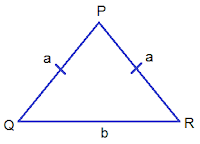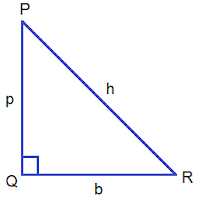# Perimeter of Triangle## Perimeter of Triangle

Perimeter is the total length of the boundary of a closed plane figure. The boundary can be circular, triangular, rectangular and so on. In other words, the sum of the lengths of sides of any closed plane figure is the perimeter of the figure.

A triangle is a closed plane figure bounded by 3 sides. So the sum of the lengths of 3 sides of a triangle is called the perimeter of triangle.
In ΔPQR, PQ = 5 cm, QR = 6 cm and PR = 7 cm. Find the perimeter of the ΔPQR.
Here,
The sum of the sides of ΔPQR = PQ + QR + PR = 5 cm + 6 cm + 7 cm = 18 cm.
The perimeter of ΔPQR is 18 cm.

###Perimeter of a Scalene Triangle

If non of the sides of a trianlge are equal it is called a scalene triangle. In such condition it is customary to write three sides of the trianlge as a, b and c. So the formula for perimeter of a scalene triangle is a+b+c.
i.e. P = a + b + c

###Perimeter of an Isosceles Triangle

If two sides of a triangle are equal it called an isosceles triangle. In such condition two equal sides are represented by a and the third side is termed as base and represented by b. So the formula for perimeter of an isosceles triangle is a+a+b.
i.e. P = a + a + b

###Perimeter of an Equilateral Triangle

If all 3 sides are equal of a triangle it is called an equilateral triangle. In such triangles we represent each equal side by a. So the formula for perimeter is a+a+a or 3a.
i.e. P = 3a

### Perimeter of a Right Angled TriangleIf one angle of a triangle is right angle then it called a right angled triangle. Three sides of a right angled triangle are termed as perpendicular (p), base (b) and hypotenuse (h). So the perimeter of a right angled triangle is p+b+h.
i.e. P = p + b + h

### Workout Examples

Example 1: Find the perimeter of the the triangle given below:
Solution:
From the figure,
a = 4 cm
b = 7 cm
c = 6 cm
we know,
Perimeter = a + b + c
= 4cm + 7cm + 6cm
= 17 cm

Example 2: Find the perimeter of the given triangle.

Solution:
Given figure is an equilateral triangle where
a = 8 cm
we know,
Perimeter = 3a
= 3 × 8 cm
= 24 cm

Example 3: Find the perimeter of the given triangle.

Solution:
Given figure is a right angled triangle where
p = 4 cm
h = 5 cm

we know,
Perimeter = p + b + h
= 4cm + 3cm + 5cm
= 12 cm

You can comment your problems/questions regarding perimeter of triangle here.